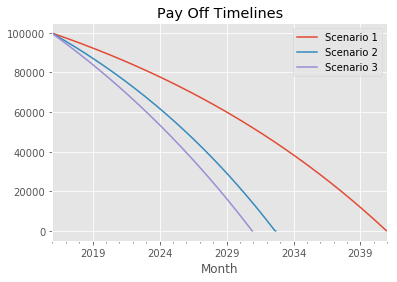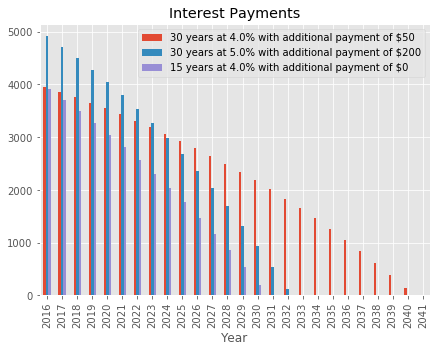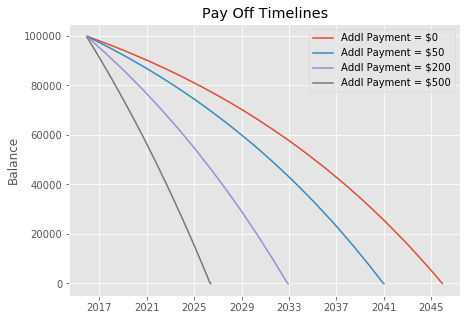Updated Notebook from Practical Business Python.

Original article had a model that did not work correctly. This notebook is for the updated article.

Many thanks to the individuals that helped me fix the errors. The solution below is based heavily on this gist and comments on reddit.

In :
import pandas as pd
from datetime import date
import numpy as np
from collections import OrderedDict
from dateutil.relativedelta import *
import matplotlib.pyplot as plt
from IPython.core.pylabtools import figsize


Build a payment schedule using a generator that can be easily read into a pandas dataframe for additional analysis and plotting

In :
def amortize(principal, interest_rate, years, pmt, addl_principal, start_date, annual_payments):
"""
Calculate the amortization schedule given the loan details.

:param principal: Amount borrowed
:param interest_rate: The annual interest rate for this loan
:param years: Number of years for the loan
:param pmt: Payment amount per period
:param start_date: Start date for the loan.
:param annual_payments: Number of payments in a year.

:return:
schedule: Amortization schedule as an Ortdered Dictionary
"""

# initialize the variables to keep track of the periods and running balances
p = 1
beg_balance = principal
end_balance = principal

while end_balance > 0:

# Recalculate the interest based on the current balance
interest = round(((interest_rate/annual_payments) * beg_balance), 2)

# Determine payment based on whether or not this period will pay off the loan
pmt = min(pmt, beg_balance + interest)
principal = pmt - interest

# Ensure additional payment gets adjusted if the loan is being paid off
end_balance = beg_balance - (principal + addl_principal)

yield OrderedDict([('Month',start_date),
('Period', p),
('Begin Balance', beg_balance),
('Payment', pmt),
('Principal', principal),
('Interest', interest),
('End Balance', end_balance)])

# Increment the counter, balance and date
p += 1
start_date += relativedelta(months=1)
beg_balance = end_balance


Wrapper function to call amortize.

This function primarily cleans up the table and provides summary stats so it is easy to compare various scenarios.

In :
def amortization_table(principal, interest_rate, years,
"""
Calculate the amortization schedule given the loan details as well as summary stats for the loan

:param principal: Amount borrowed
:param interest_rate: The annual interest rate for this loan
:param years: Number of years for the loan

:param annual_payments (optional): Number of payments in a year. DEfault 12.
:param start_date (optional): Start date. Default first of next month if none provided

:return:
schedule: Amortization schedule as a pandas dataframe
summary: Pandas dataframe that summarizes the payoff information
"""

# Payment stays constant based on the original terms of the loan
payment = -round(np.pmt(interest_rate/annual_payments, years*annual_payments, principal), 2)

# Generate the schedule and order the resulting columns for convenience
schedule = pd.DataFrame(amortize(principal, interest_rate, years, payment,
schedule = schedule[["Period", "Month", "Begin Balance", "Payment", "Interest",

# Convert to a datetime object to make subsequent calcs easier
schedule["Month"] = pd.to_datetime(schedule["Month"])

#Create a summary statistics table
payoff_date = schedule["Month"].iloc[-1]
stats = pd.Series([payoff_date, schedule["Period"].count(), interest_rate,
schedule["Interest"].sum()],
index=["Payoff Date", "Num Payments", "Interest Rate", "Years", "Principal",

return schedule, stats


Example showing how to call the function

In :
df, stats = amortization_table(700000, .04, 30, addl_principal=200, start_date=date(2016, 1,1))

In :
stats

Out:
Payoff Date           2042-12-01 00:00:00
Num Payments                          324
Interest Rate                        0.04
Years                                  30
Principal                          700000
Payment                           3341.91
Total Interest                     444406
dtype: object
In :
df.head()

Out:
Period Month Begin Balance Payment Interest Principal Additional_Payment End Balance
0 1 2016-01-01 700000.00 3341.91 2333.33 1008.58 200.0 698791.42
1 2 2016-02-01 698791.42 3341.91 2329.30 1012.61 200.0 697578.81
2 3 2016-03-01 697578.81 3341.91 2325.26 1016.65 200.0 696362.16
3 4 2016-04-01 696362.16 3341.91 2321.21 1020.70 200.0 695141.46
4 5 2016-05-01 695141.46 3341.91 2317.14 1024.77 200.0 693916.69
In :
df.tail()

Out:
Period Month Begin Balance Payment Interest Principal Additional_Payment End Balance
319 320 2042-08-01 14413.65 3341.91 48.05 3293.86 200.0 10919.79
320 321 2042-09-01 10919.79 3341.91 36.40 3305.51 200.0 7414.28
321 322 2042-10-01 7414.28 3341.91 24.71 3317.20 200.0 3897.08
322 323 2042-11-01 3897.08 3341.91 12.99 3328.92 200.0 368.16
323 324 2042-12-01 368.16 369.39 1.23 368.16 0.0 0.00

Make multiple calls to compare scenarios

In :
schedule1, stats1 = amortization_table(100000, .04, 30, addl_principal=50, start_date=date(2016,1,1))
schedule2, stats2 = amortization_table(100000, .05, 30, addl_principal=200, start_date=date(2016,1,1))
schedule3, stats3 = amortization_table(100000, .04, 15, addl_principal=0, start_date=date(2016,1,1))

In :
pd.DataFrame([stats1, stats2, stats3])

Out:
Payoff Date Num Payments Interest Rate Years Principal Payment Additional Payment Total Interest
0 2041-01-01 301 0.04 30 100000 477.42 50 58441.08
1 2032-09-01 201 0.05 30 100000 536.82 200 47708.38
2 2030-12-01 180 0.04 15 100000 739.69 0 33143.79

Make some plots to show scenarios

In :
%matplotlib inline
plt.style.use('ggplot')

In :
fig, ax = plt.subplots(1, 1)
schedule1.plot(x='Month', y='End Balance', label="Scenario 1", ax=ax)
schedule2.plot(x='Month', y='End Balance', label="Scenario 2", ax=ax)
schedule3.plot(x='Month', y='End Balance', label="Scenario 3", ax=ax)
plt.title("Pay Off Timelines");In :
def make_plot_data(schedule, stats):
"""Create a dataframe with annual interest totals, and a descriptive label"""
y = schedule.set_index('Month')['Interest'].resample("A").sum().reset_index()
y["Year"] = y["Month"].dt.year
y.set_index('Year', inplace=True)
y.drop('Month', 1, inplace=True)
label="{} years at {}% with additional payment of ${}".format(stats['Years'], stats['Interest Rate']*100, stats['Additional Payment']) return y, label y1, label1 = make_plot_data(schedule1, stats1) y2, label2 = make_plot_data(schedule2, stats2) y3, label3 = make_plot_data(schedule3, stats3) y = pd.concat([y1, y2, y3], axis=1)  In : figsize(7,5) fig, ax = plt.subplots(1, 1) y.plot(kind="bar", ax=ax) plt.legend([label1, label2, label3], loc=1, prop={'size':10}) plt.title("Interest Payments");In : additional_payments = [0, 50, 200, 500] fig, ax = plt.subplots(1, 1) for pmt in additional_payments: result, _ = amortization_table(100000, .04, 30, addl_principal=pmt, start_date=date(2016,1,1)) ax.plot(result['Month'], result['End Balance'], label='Addl Payment =${}'.format(str(pmt)))
plt.title("Pay Off Timelines")
plt.ylabel("Balance")
ax.legend();In [ ]: